Tags: tutorial connectivity

# Whole brain connectivity and network analysis

## Introduction

This tutorial will demonstrate one of the possible ways to analyze MEG data from a graph theoretical perspective. It is assumed that you are familiar with the various preprocessing steps which will be performed here, as these are not explained further in detail. An overview and detailed information on preprocessing can be found here and on time-frequency analysis here

This tutorial will use metrics that are derived from graph theory and are implemented in the Brain Connectivity Toolbox (BCT, detailed explanation can be found here).

## Background

We will analyze brain signals acquired during a resting condition with eyes closed. In contrast to an event-related analysis, where the data is segmented around some external events, we will analyze the entire continuous recording of approximately 5 minutes length. We will compute connectomes that quantify the ‘connectivity’ between all pairs of pre defined regions, adopting a parcellation approach.

Such connectomes are typically used for a subsequent graph analysis, to extract specific structure in the connections. We will illustrate this by visualizing the node degree.

Also, we will explore the connectomes in more detail, and investigate how the spatial structure changes when moving from one seed location to another.

## Procedure

The data analyses will follow the following steps:

## Preprocessing

The aim is to identify the frequency and topography of an 10Hz oscillation. We first use ft_preprocessing to read the continuous data and

ft_redefinetrial to segment it into epochs of 2 seconds length.

The ft_redefinetrial and ft_preprocessing functions require the original MEG dataset, which is available from https://download.fieldtriptoolbox.org/tutorial/SubjectRest.zip. Alternatively, you can skip this step and directly load the preprocessed data from https://download.fieldtriptoolbox.org/tutorial/networkanalysis/. This latter folder contains a few files that we will need later in this tutorial as well, so it is recommended to download its contents.

``````%% read the continuous data and segment into 2 seconds epochs
cfg            = [];
cfg.dataset    = 'SubjectRest.ds'; % note that you may need to add the full path to the .ds directory
cfg.continuous = 'yes';
cfg.channel    = {'MEG'};
data           = ft_preprocessing(cfg);

cfg         = [];
cfg.length  = 2;
cfg.overlap = 0.5;
data        = ft_redefinetrial(cfg, data);

% this step is needed to 1) remove the DC-component, and to 2) get rid of a few segments of data at
% the end of the recording, which contains only 0's.
cfg        = [];
cfg.demean = 'yes';
cfg.trials = 1:(numel(data.trial)-6);
data       = ft_preprocessing(cfg, data);
``````

### Artefact rejection

We will first clean the data from potential bad segments such as SQUID jumps and/or bad channels using ft_rejectvisual. Subsequently, we will identify occular and cardiac artifacts by means of ICA using ft_componentanalysis. Since, these type of artifacts are predominately low frequent and we are interested in a 10Hz signal, we will downsample the data using ft_resampledata in order to speed up calculations during ft_componentanalysis and reduce potential working memory issues. Alternatively, you can skip these steps and download the data here.

``````%% make a visual inspection and reject bad trials/sensors
cfg         = [];
cfg.method  = 'summary';
cfg.channel = 'MEG';
cfg.layout  = 'CTF275.lay';
dataclean   = ft_rejectvisual(cfg, data);

%% you can identify the rejected trial numbers by typing
trlind = [];
for i=1:length(dataclean.cfg.artfctdef.summary.artifact)
end

% alternatively, you can use the list below, this is the definition of the badtrials for the data that has been stored on dis
%badtrials  = [18 19 21 72 73 74 75 76 93 94 109 110 126 127 128 140 172 173 179 180 181 182 196 197 198 227 228 233 243 244 250 251 265 266 286];

cfg        = [];
dataclean  = ft_selectdata(cfg, data);

%% downsample the data to speed up component analysis
dataclean.time(1:end) = dataclean.time(1); % this avoids numeric round off issues in the time axes upon resampling

cfg            = [];
cfg.resamplefs = 100;
cfg.detrend    = 'yes';

%% use ICA in order to identify cardiac and blink components
cfg                 = [];
cfg.method          = 'runica';
cfg.runica.maxsteps = 50;
%cfg.randomseed      = 0; % this can be uncommented to match the data that has been stored on disk

%% visualize components

% these were the indices of the bad components that were identified
% they may be different if you re-run the ICA decomposition with a random randomseed.
badcomp = [2 3 7 16];

cfg            = [];
cfg.layout     = 'CTF275_helmet.mat';
cfg.compscale  = 'local';
cfg.continuous = 'yes';
ft_databrowser(cfg, comp);

cfg           = [];
dataica       = ft_rejectcomponent(cfg, comp);
``````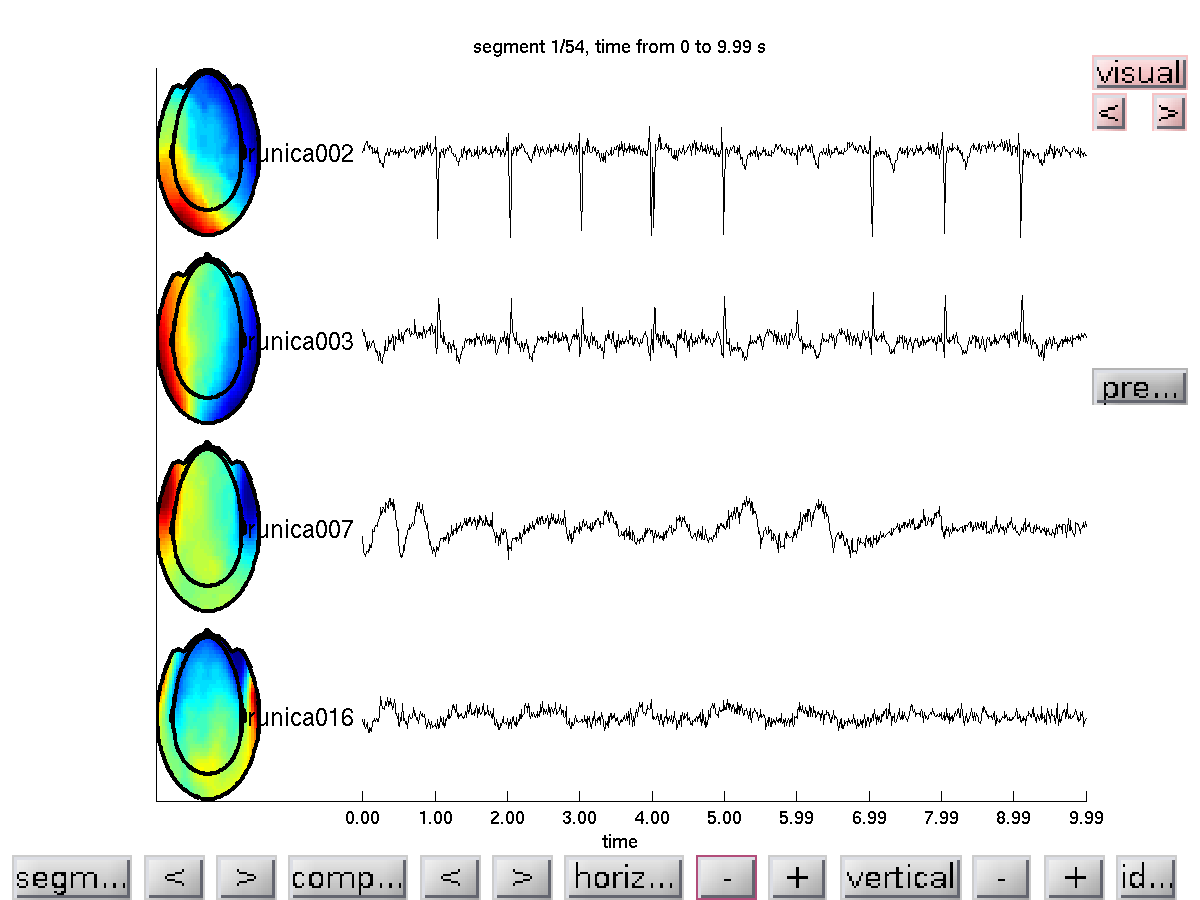Figure 1: Topography and time course of IC’s likely reflecting cardiac and eye movement artifacts

We project the component data back to the channel representation, leaving out the bad components.

``````cfg            = [];
dataica        = ft_rejectcomponent(cfg, comp);
``````

### Spectral analysis

We will analyze the spectral content of the data using ft_freqanalysis and subsequently interactively explore the data with ft_topoplotER and ft_singleplotER. For those interested in more detailed overview of the configuration options and strategies please refer to our video lectures here and also here.

``````%% compute the power spectrum
cfg              = [];
cfg.output       = 'pow';
cfg.method       = 'mtmfft';
cfg.taper        = 'dpss';
cfg.tapsmofrq    = 1;
cfg.keeptrials   = 'no';
datapow          = ft_freqanalysis(cfg, dataica);

%% compute the planar transformation, this is not really necessary, but instructive anyhow

dataicatmp      = dataica;

cfg               = [];
cfg.neighbours    = neighbours;
cfg.planarmethod  = 'sincos';
planar            = ft_megplanar(cfg, dataicatmp);
clear dataicatmp;

%% compute the power spectrum
cfg              = [];
cfg.output       = 'pow';
cfg.method       = 'mtmfft';
cfg.taper        = 'dpss';
cfg.tapsmofrq    = 1;
cfg.keeptrials   = 'no';
datapow_planar   = ft_freqanalysis(cfg, planar);

%% plot the topography and the spectrum
figure;

cfg        = [];
cfg.layout = 'CTF275_helmet.mat';
cfg.xlim   = [9 11];
subplot(2,2,1); ft_topoplotER(cfg, datapow);
subplot(2,2,2); ft_topoplotER(cfg, ft_combineplanar([], datapow_planar));

cfg         = [];
cfg.channel = {'MRO22', 'MRO32', 'MRO33'};
subplot(2,2,3); ft_singleplotER(cfg, datapow);
``````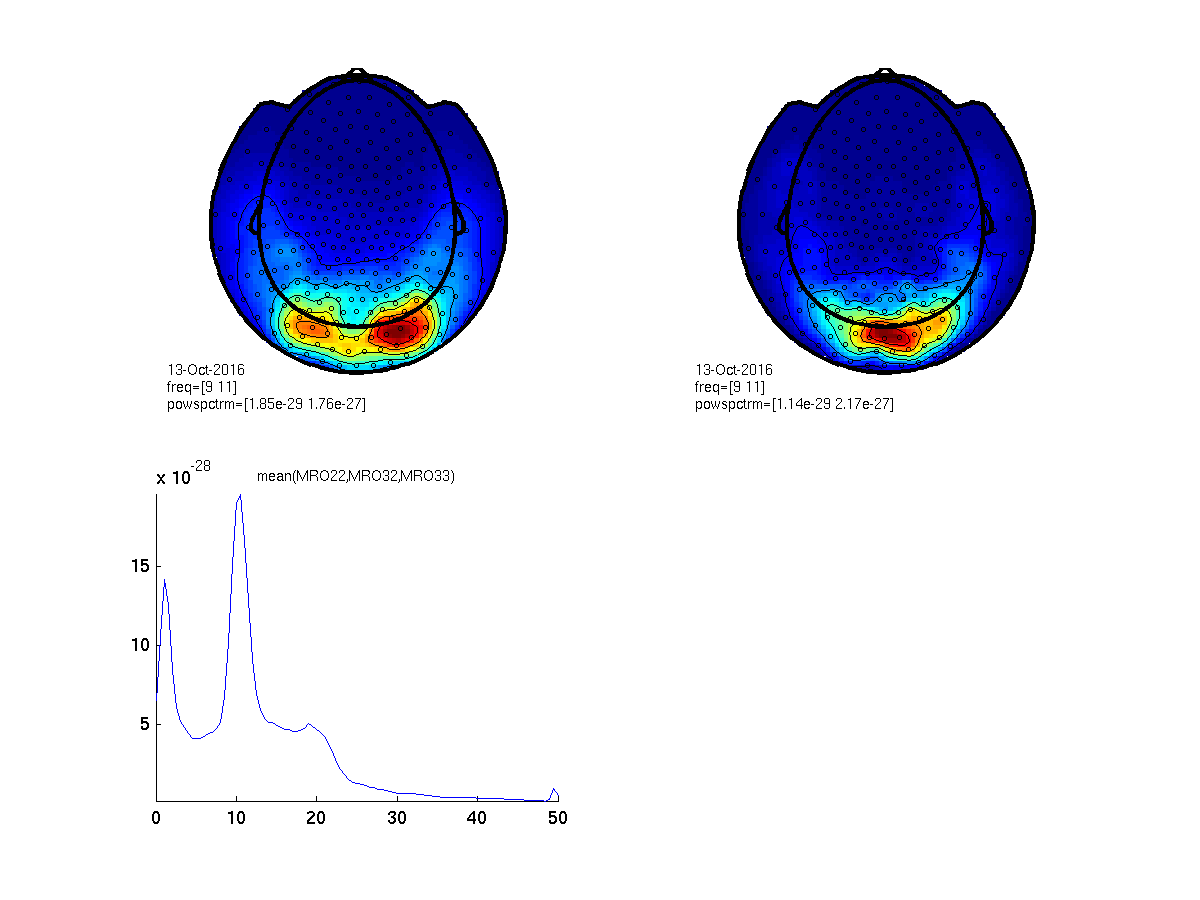Figure 2: Top- scalp topography of oscillatory power centered at 10 Hz (left: axial gradient representation, right: planar gradient representation). Bottom- power spectrum averaged over three occipital sensors illustrating a clear ~10 Hz peak.

### Computation of the forward model

In the following section we will compute the forward model, i.e. the leadfield matrix that defines for a set of predefined dipole locations the expected magnetic field distribution as it is picked up by the MEG sensors. In this tutorial we will use a cortical sheet based source model, in which the individual dipole locations are constrained to the cortical sheet. This anatomical model has been obtained with freesurfer and it takes quite some time to generate. This falls outside the scope of this tutorial. If you would like to get an idea how this can be done, please have a look at our sourcemodel tutorial. Alternatively, one could create a volumetric dipole grid based on regularly spaced 3-dimensional grid of dipole locations, or an inverse-warp from MNI normalized volumetric space of a template 3D grid. More information about this can be found in our sourcemodel tutorial as well.

``````%% load the required geometrical information

%% visualize the coregistration of sensors, headmodel, and sourcemodel.
figure;

% make the headmodel surface transparent
ft_plot_mesh(ft_convert_units(sourcemodel, 'cm'),'vertexcolor',sourcemodel.sulc);
view([0 -90 0])
``````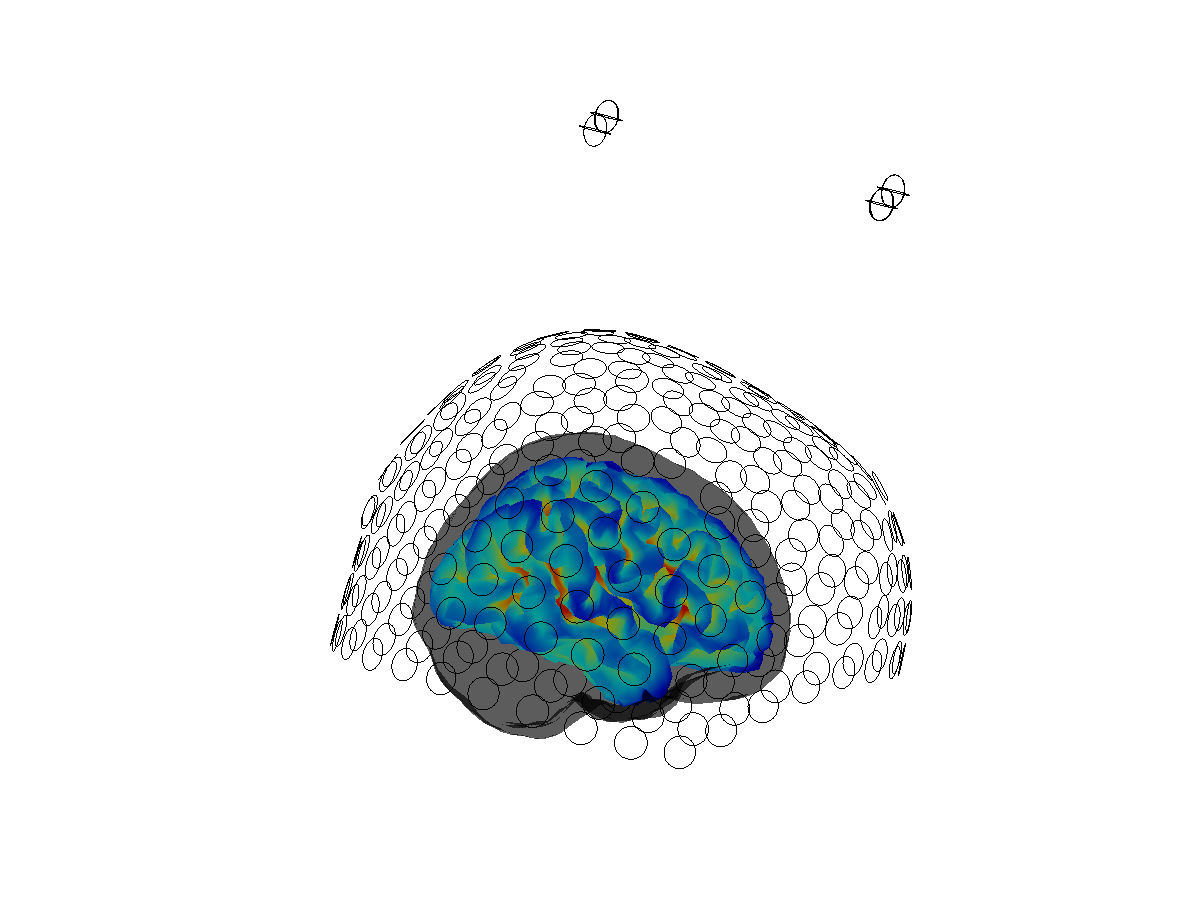Figure 3: Coregistration between headmodel, sourcemodel and sensor array.

Now we can proceed with the computation of the leadfield matrix, using ft_prepare_leadfield.

``````%% compute the leadfield
cfg             = [];
cfg.grid        = sourcemodel;
cfg.channel     = {'MEG'};
``````

### Source reconstruction

In addition to a forward model, the beamformer needs a sensor-level covariance matrix, or a cross-spectral density matrix. The preliminaries for the cross-spectral density matrix can be obtained with

ft_freqanalysis. In this tutorial, you will compute a memory-wise more compact representation of the single epoch spectral representation, from which the cross-spectral density can be computed in a straightforward way. This will be done ‘under the hood’ in ft_sourceanalysis, so you don’t need to worry about this particular conversion step.

``````%% compute sensor level Fourier spectra, to be used for cross-spectral density computation.
cfg            = [];
cfg.method     = 'mtmfft';
cfg.output     = 'fourier';
cfg.keeptrials = 'yes';
cfg.tapsmofrq  = 1;
cfg.foi        = 10;
freq           = ft_freqanalysis(cfg, dataica);
``````

Next, we call ft_sourceanalysis with ‘pcc’ as method. Essentially, this methods implements DICS (the underlying algorithm for computing the spatial filters is according to DICS), but provides more flexibility with respect to data handling. In this context, the advantage is that the ‘pcc’-implementation directly outputs, for each dipole location in the sourcemodel, the fourier coefficients (i.e. phase and amplitude estimates) for each of the trials. This can subsequently be used in a straightforward way for connectivity analysis. In contrast, using ‘dics’ as a method, to obtain the single trial representation of phase and amplitude is quite a bit more tedious.

``````%% do the source reconstruction
cfg                   = [];
cfg.frequency         = freq.freq;
cfg.method            = 'pcc';
cfg.grid              = lf;
cfg.keeptrials        = 'yes';
cfg.pcc.lambda        = '10%';
cfg.pcc.projectnoise  = 'yes';
cfg.pcc.fixedori      = 'yes';
source = ft_sourceanalysis(cfg, freq);
source = ft_sourcedescriptives([], source); % to get the neural-activity-index
``````

### Visualization of the neural-activity-index

In order to visualize source-reconstructed data, the function ft_sourceplot can be used. If the input data contains the dipole positions defined on a triangulated mesh (i.e. it contains both a ‘pos’ and a ‘tri’ field), one should use the ‘surface’ method.

``````%% plot the neural activity index (power/noise)
cfg               = [];
cfg.method        = 'surface';
cfg.funparameter  = 'nai';
cfg.funcolorlim   = [0.0 8];
cfg.opacitylim    = [3 8];
cfg.opacitymap    = 'rampup';
cfg.funcolormap   = 'jet';
cfg.colorbar      = 'no';
ft_sourceplot(cfg, source);
view([-90 30]);
light;
``````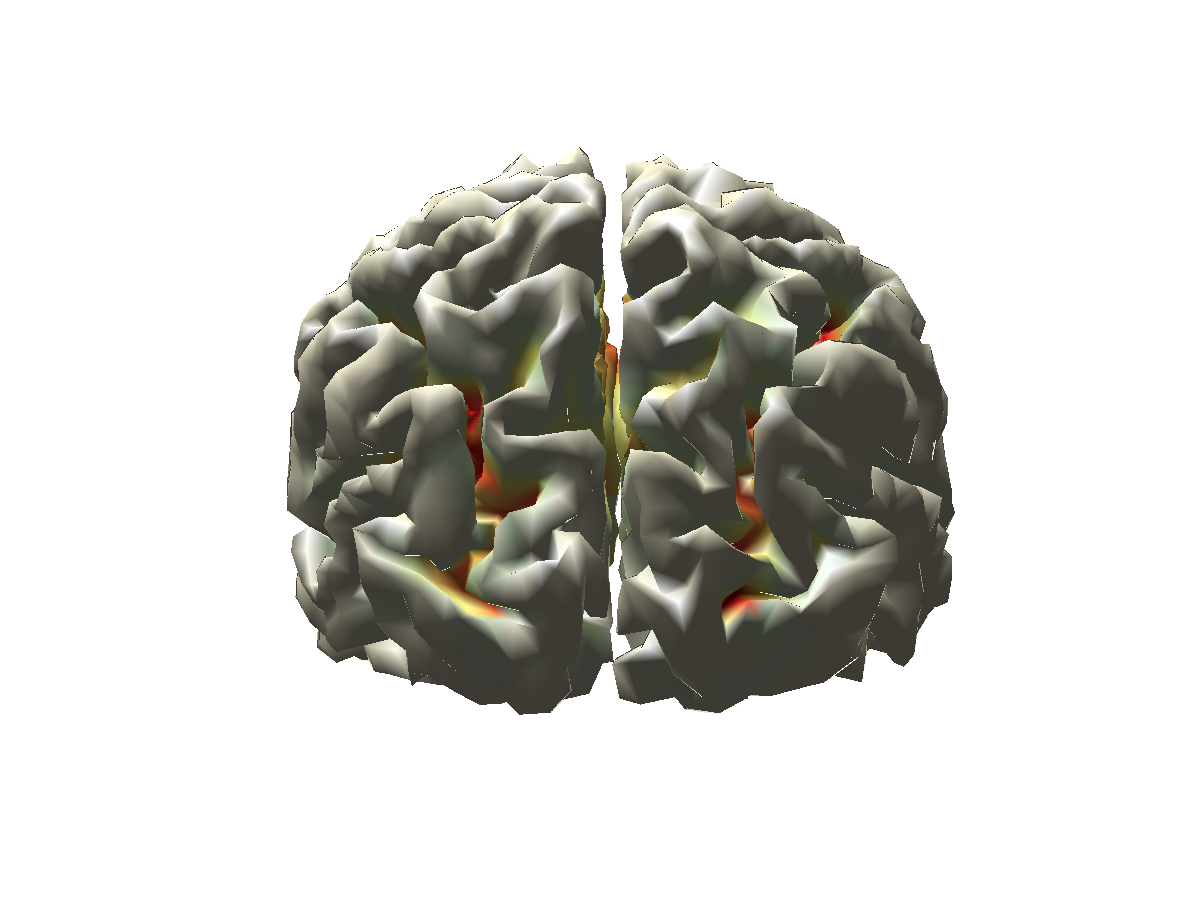Figure 4: Reconstructed activity (neural activity index) of resting state alpha power is not as instructive as one would hope.

Compare the distribution of the neural activity index with the sensor topographies plotted earlier. How do they compare? Could you give an explanation of why the correspondence could be poor?

### Creation of a ‘pseudo-contrast’ based on a median split of the epochs

Typically, in an experimental context, it is useful to visualize activity contrasts, e.g., baseline vs. activation intervals, in order to get spatially interpretable beamformer results. Although the neural-activity-index intends to improve interpretability by normalization with a poor man’s approximation of the projected noise, and although it takes care of the depth bias of the beamformer to some extent, it doesn’t usually work well. In order to convince ourselves that the beamformer is adequately reconstructing the activity of the neural sources, we will resort here to faking an ‘experimental’ contrast, using a median split of the data, where the data are split according to occipital alpha power. This requires an estimate of the single epoch alpha power. Next, identify the epoch indices for which the alpha power is less/more than the median across epochs.

``````%% compute sensor level single trial power spectra
cfg              = [];
cfg.output       = 'pow';
cfg.method       = 'mtmfft';
cfg.taper        = 'dpss';
cfg.foilim       = [9 11];
cfg.tapsmofrq    = 1;
cfg.keeptrials   = 'yes';
datapow           = ft_freqanalysis(cfg, dataica);

%% identify the indices of trials with high and low alpha power
freqind = nearest(datapow.freq, 10);
tmp     = datapow.powspctrm(:,:,freqind);
chanind = find(mean(tmp,1)==max(mean(tmp,1)));  % find the sensor where power is max
indlow  = find(tmp(:,chanind)<=median(tmp(:,chanind)));
indhigh = find(tmp(:,chanind)>=median(tmp(:,chanind)));
``````

Now, we can compute the spectra for the two sets of epochs using ft_freqdescriptives and compute the difference with ft_math

``````%% compute the power spectrum for the median splitted data
cfg              = [];
cfg.trials       = indlow;
datapow_low      = ft_freqdescriptives(cfg, datapow);

cfg.trials       = indhigh;
datapow_high     = ft_freqdescriptives(cfg, datapow);

%% compute the difference between high and low
cfg = [];
cfg.parameter = 'powspctrm';
cfg.operation = 'divide';
powratio      = ft_math(cfg, datapow_high, datapow_low);

%% plot the topography of the difference along with the spectra
cfg        = [];
cfg.layout = 'CTF275_helmet.mat';
cfg.xlim   = [9.9 10.1];
figure; ft_topoplotER(cfg, powratio);

cfg         = [];
cfg.channel = {'MRO33'};
figure; ft_singleplotER(cfg, datapow_high, datapow_low);
``````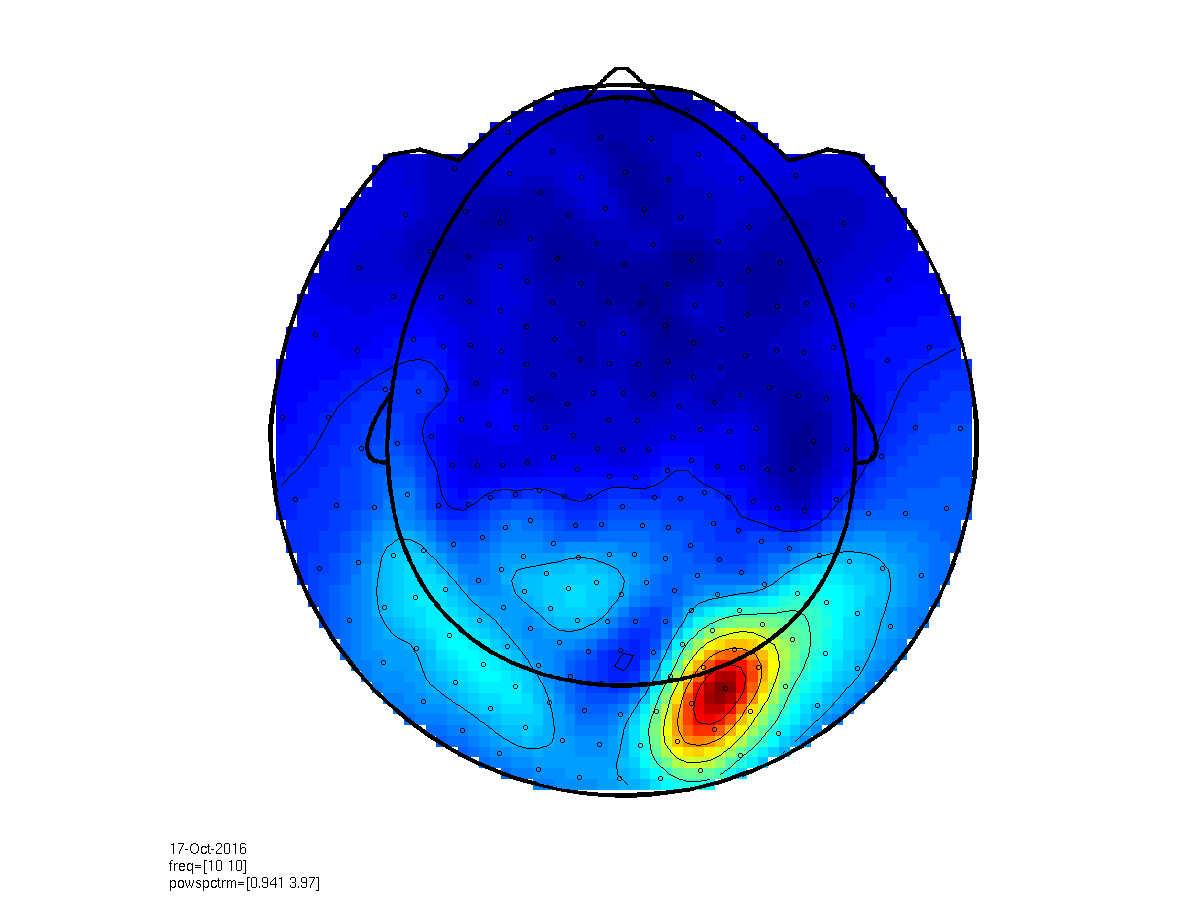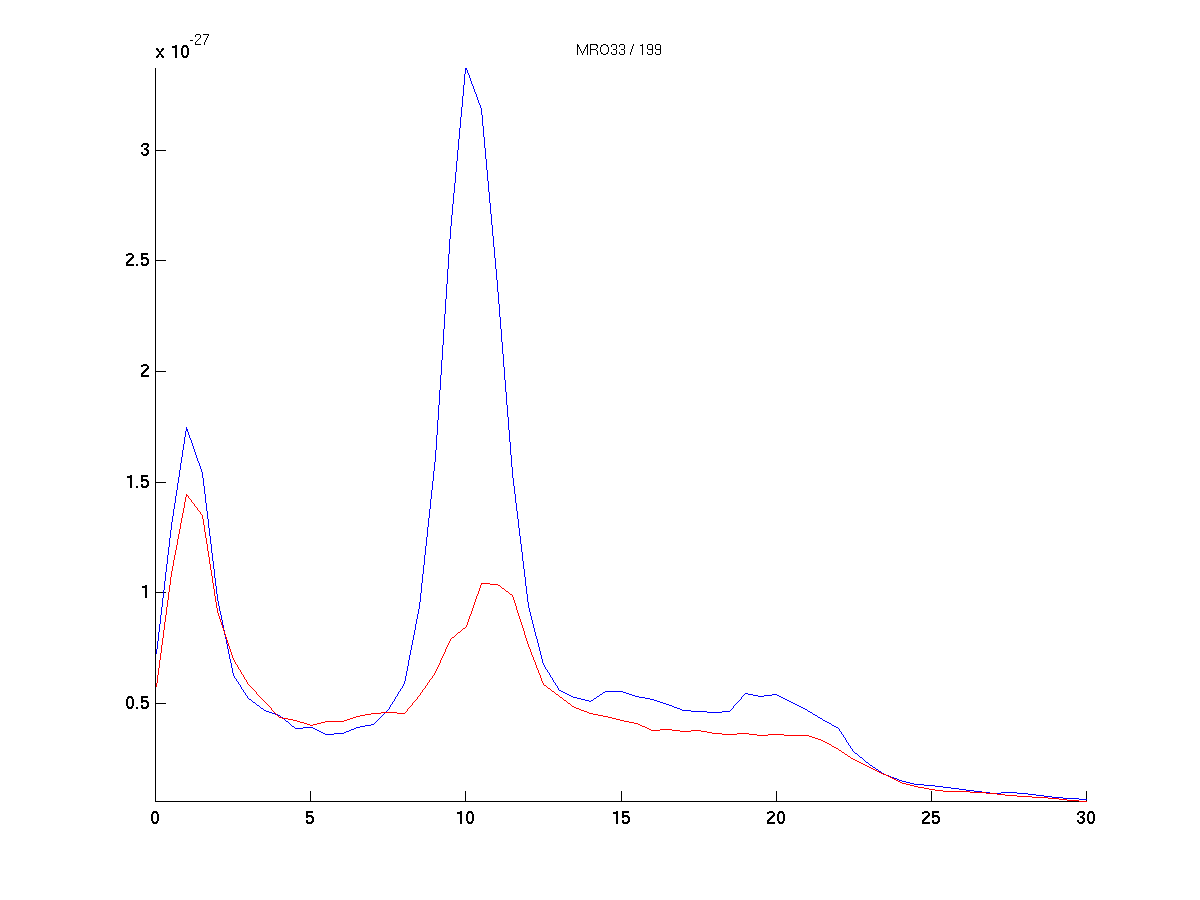Figure 5: Difference topography (left) and power spectra of the median splitted data, according to 10 Hz power at sensor ‘MRO33’.

### Source reconstruction of ‘low’ and ‘high’ alpha activity epochs

Now we will compute the source reconstructed alpha power again, as illustrated above, based on the median split. We will use a common filter approach, where we compute the spatial filters based on the cross-spectral density averaged across all epochs. See also here and here for further information on common filters.

``````%% compute fourier spectra for frequency of interest according to the trial split
cfg            = [];
cfg.method     = 'mtmfft';
cfg.output     = 'fourier';
cfg.keeptrials = 'yes';
cfg.tapsmofrq  = 1;
cfg.foi        = 10;

cfg.trials = indlow;
freq_low   = ft_freqanalysis(cfg, dataica);

cfg.trials = indhigh;
freq_high  = ft_freqanalysis(cfg, dataica);

%% compute the beamformer filters based on the entire data
cfg                   = [];
cfg.frequency         = freq.freq;
cfg.method            = 'pcc';
cfg.grid              = lf;
cfg.keeptrials        = 'yes';
cfg.pcc.lambda        = '10%';
cfg.pcc.projectnoise  = 'yes';
cfg.pcc.keepfilter    = 'yes';
cfg.pcc.fixedori      = 'yes';
source = ft_sourceanalysis(cfg, freq);

% use the precomputed filters
cfg                   = [];
cfg.frequency         = freq.freq;
cfg.method            = 'pcc';
cfg.grid              = lf;
cfg.sourcemodel.filter       = source.avg.filter;
cfg.keeptrials        = 'yes';
cfg.pcc.lambda        = '10%';
cfg.pcc.projectnoise  = 'yes';
source_low  = ft_sourcedescriptives([], ft_sourceanalysis(cfg, freq_low));
source_high = ft_sourcedescriptives([], ft_sourceanalysis(cfg, freq_high));

cfg           = [];
cfg.operation = 'log10(x1)-log10(x2)';
cfg.parameter = 'pow';
source_ratio  = ft_math(cfg, source_high, source_low);
``````

We now create a fancy opacity mask for the functional data, and visualize the log-difference on the cortical sheet.

``````% create a fancy mask

cfg = [];
cfg.method        = 'surface';
cfg.funparameter  = 'pow';
cfg.funcolorlim   = [-.3 .3];
cfg.funcolormap   = 'jet';
cfg.colorbar      = 'no';
ft_sourceplot(cfg, source_ratio);
view([-90 30]);
light('style','infinite','position',[0 -200 200]);
``````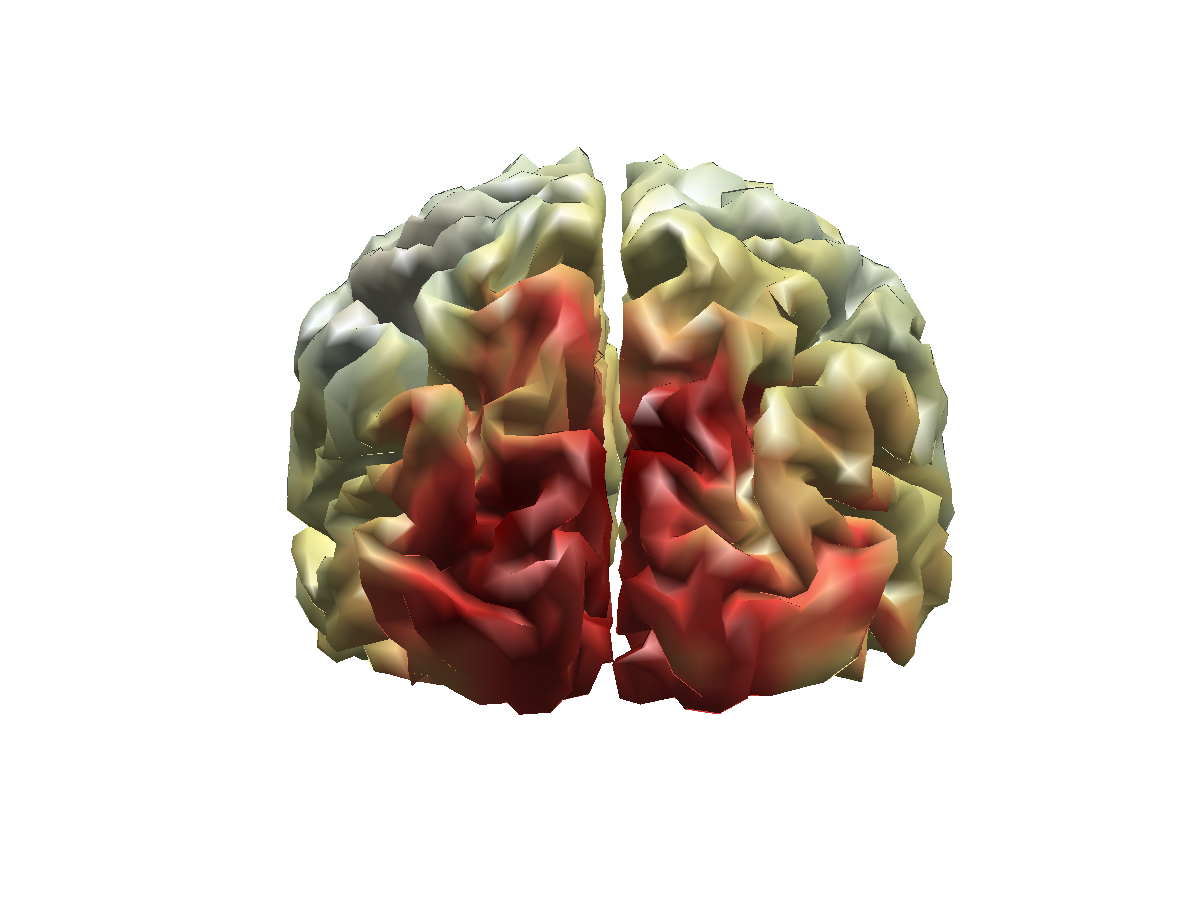Figure 6: Source reconstructed activity illustrating the relative difference in alpha power between the high and low alpha conditions.

Compare this source reconstruction with the sensor topographies generated above. How do the two representations compare?

### Connectivity analysis and parcellation

Next, we will call ft_connectivityanalysis to compute a connectivity matrix between all pairs of dipoles, which is sometimes referred to as a ‘connectome’. There are several connectivity measures to choose from. Here, we first will compute the imaginary part of the coherencey, using cfg.method = ‘coh’; and cfg.complex = ‘absimag’;. This syntax will return only the imaginary part of the coherence spectrum and effectivly suppress spurious coherence driven by electromagnetic field spread (Nolte et al. Identifying true brain interaction from EEG data using the imaginary part of coherence. Clinical Neurophysiology, 2004; 115; 2292-2307). For the computation, we take advantage of the fact that the ‘source’ variable constructed earlier, contains the single trial estimates of amplitude and phase at the source-level. This is the consequence of the fact that we used cfg.method=’pcc’ for ft_sourceanalysis, and requested cfg.output = ‘fourier’ for ft_freqanalysis.

``````%% compute connectivity
cfg         = [];
cfg.method  ='coh';
cfg.complex = 'absimag';
source_conn = ft_connectivityanalysis(cfg, source);
``````

We can now make a, rather uninformative, visualization of the connectome, plotting the full weighted graph, between all pairs of nodes.

``````figure;imagesc(source_conn.cohspctrm);
``````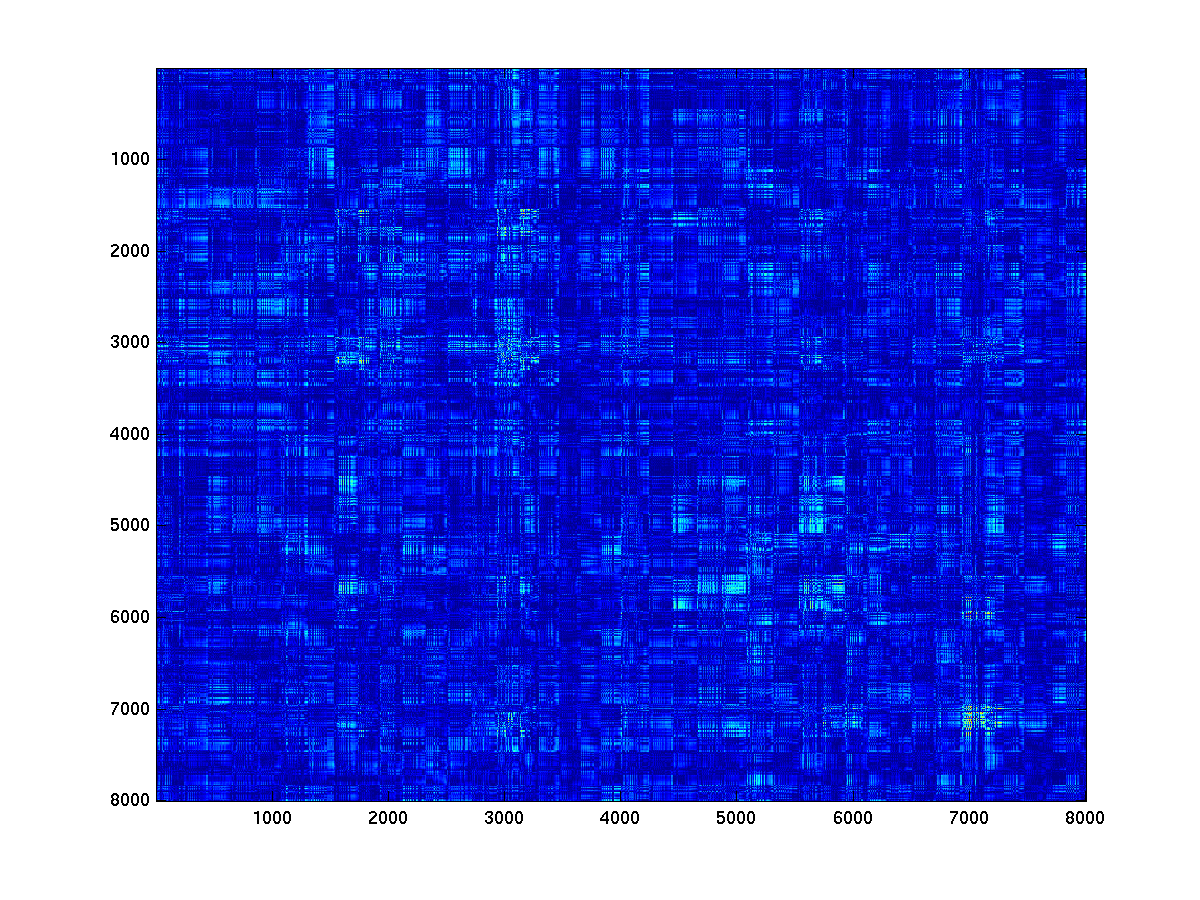Figure 7: connectivity matrix between all pairs of dipole locations

In the present example, the resulting connectivity matrix has ~64 million elements, which obviously is a very large number which does not really make sense in light of what we know about the spatial resolution of MEG. In other words, it would be a bit silly to assume each dipole locations to represent an independent neural source, and each edge to represent a separate neural connections. Therefore, one strategy to reduce the dimensionality in the data is to adopt a parcellation scheme.

When creating a parcellated connectivity matrix, we combine the connectivity values between sets of dipole pairs that belong to a given pair of parcels. Although it’s not clear what the most optimal parcellation scheme would be for MEG source reconstructed data, we could choose for a parcellation based on anatomy, e.g., using the labeling according to Brodmann. In this tutorial, we will use a parcellation that has been obtained using a multimodal parcellation scheme, and which is described in more detail here.

In fieldtrip, we use ft_sourceparcellate

``````load atlas_MMP1.0_4k.mat;
atlas.pos = source_conn.pos; % otherwise the parcellation won't work

cfg = [];
cfg.parcellation = 'parcellation';
cfg.parameter    = 'cohspctrm';
parc_conn = ft_sourceparcellate(cfg, source_conn, atlas);

figure;imagesc(parc_conn.cohspctrm);
``````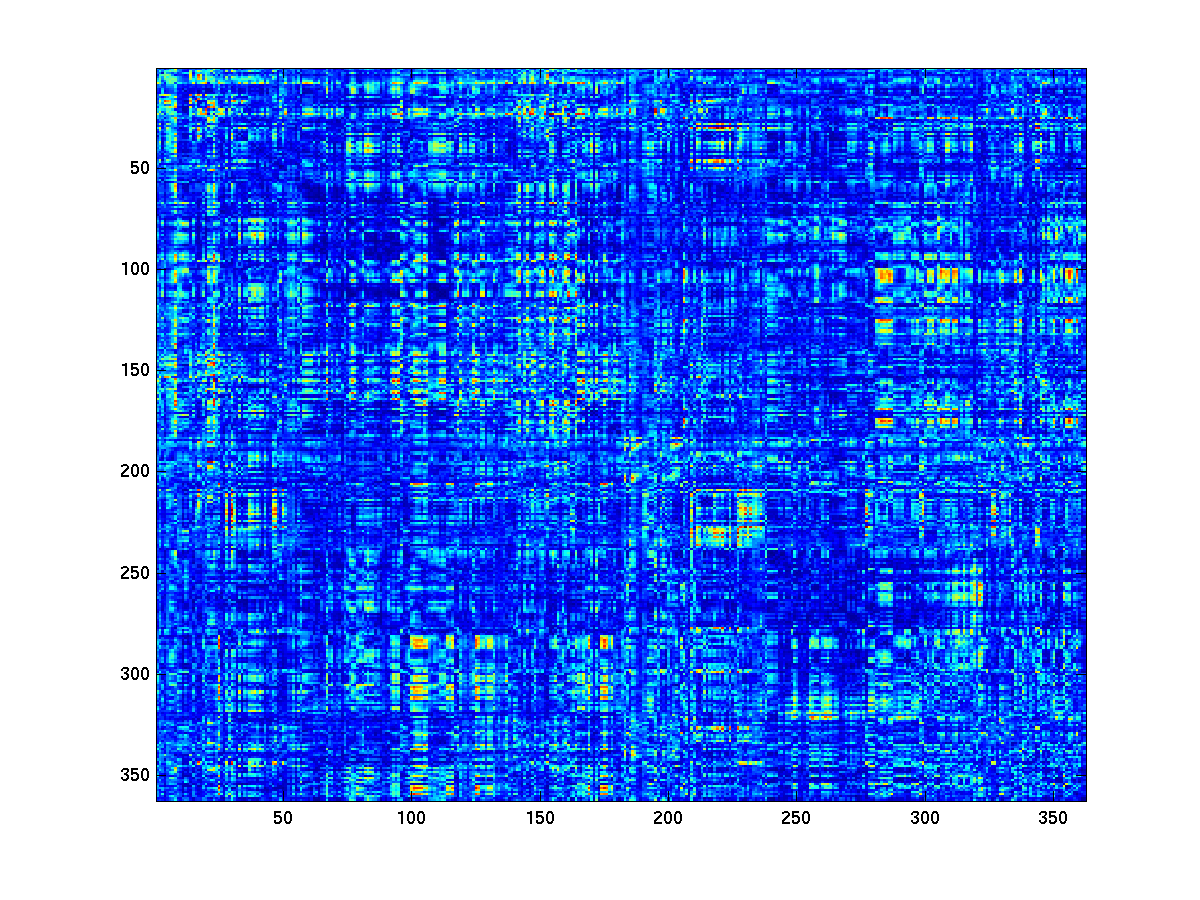Figure 7: connectivity matrix between all pairs of parcels

### Network analysis

We can now explore the structure in the estimated connectivity matrices using graph theoretic tools. It is not really clear what the effect of the residual spatial leakage of activity is on the estimates of some of these measures, so we would caution for careful interpretations of graph metrics derived from such connectivity matrices, particularly when comparing groups of experimental participants or experimental conditions. Yet, the intention of this tutorial is still to illustrate how such graph theoretic measures can in principle be computed and visualized using fieldtrip. To this end, we are going to use ft_networkanalysis, using cfg.method = ‘degrees’. Specifying a prior threshold (e.g., cfg.threshold = .1) results in an estimate of the ‘node degree’, i.e. the amount of nodes with which a particular node has an estimated connectivity of (in this case) 0.1 or higher. There are several ways to determine the threshold, for instance based on some statistical parameterization or previous observation in the literature, yet all of them are and remain arbitrary.

``````cfg           = [];
cfg.method    = 'degrees';
cfg.parameter = 'cohspctrm';
cfg.threshold = .1;
network_full = ft_networkanalysis(cfg,source_conn);
network_parc = ft_networkanalysis(cfg,parc_conn);

%% visualize
cfg               = [];
cfg.method        = 'surface';
cfg.funparameter  = 'degrees';
cfg.funcolormap   = 'jet';
ft_sourceplot(cfg, network_full);
view([-150 30]);

ft_sourceplot(cfg, network_parc);
view([-150 30]);
``````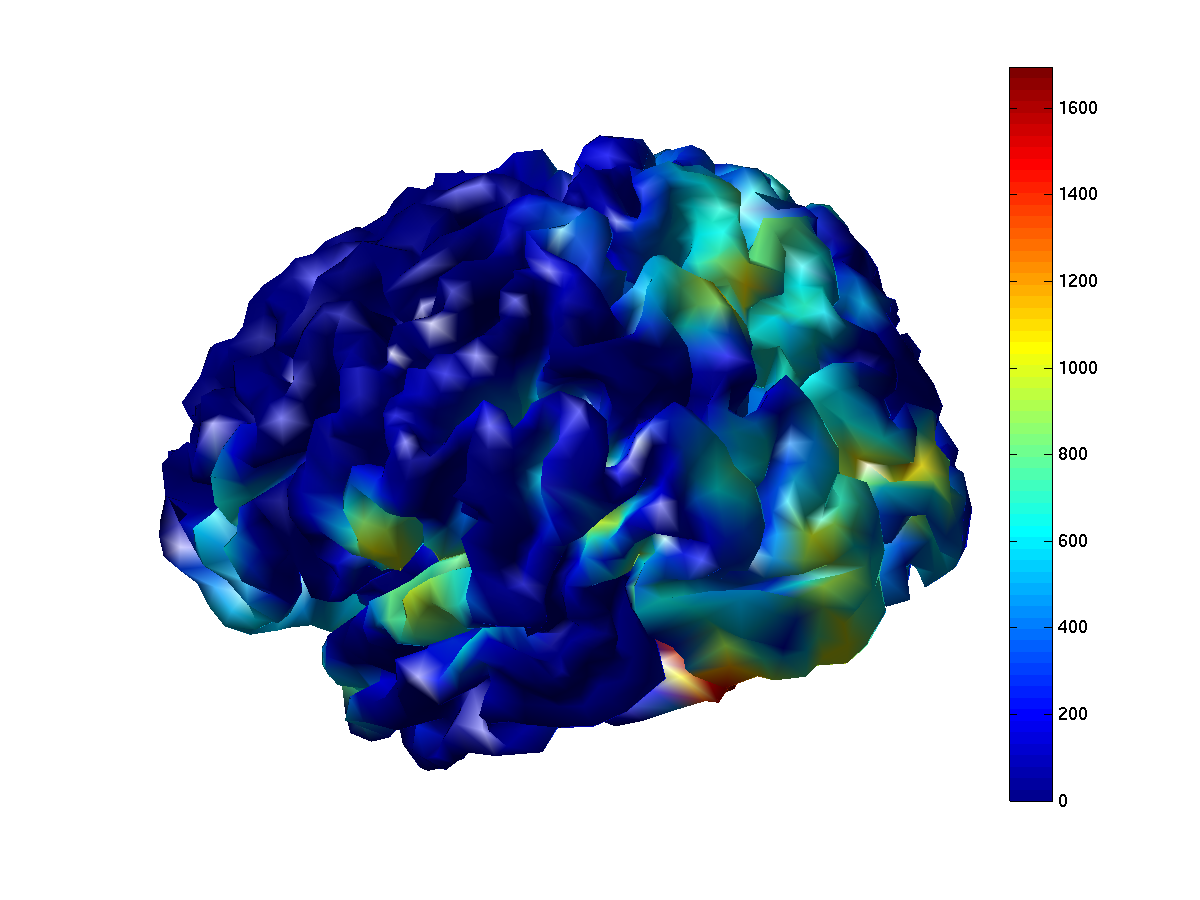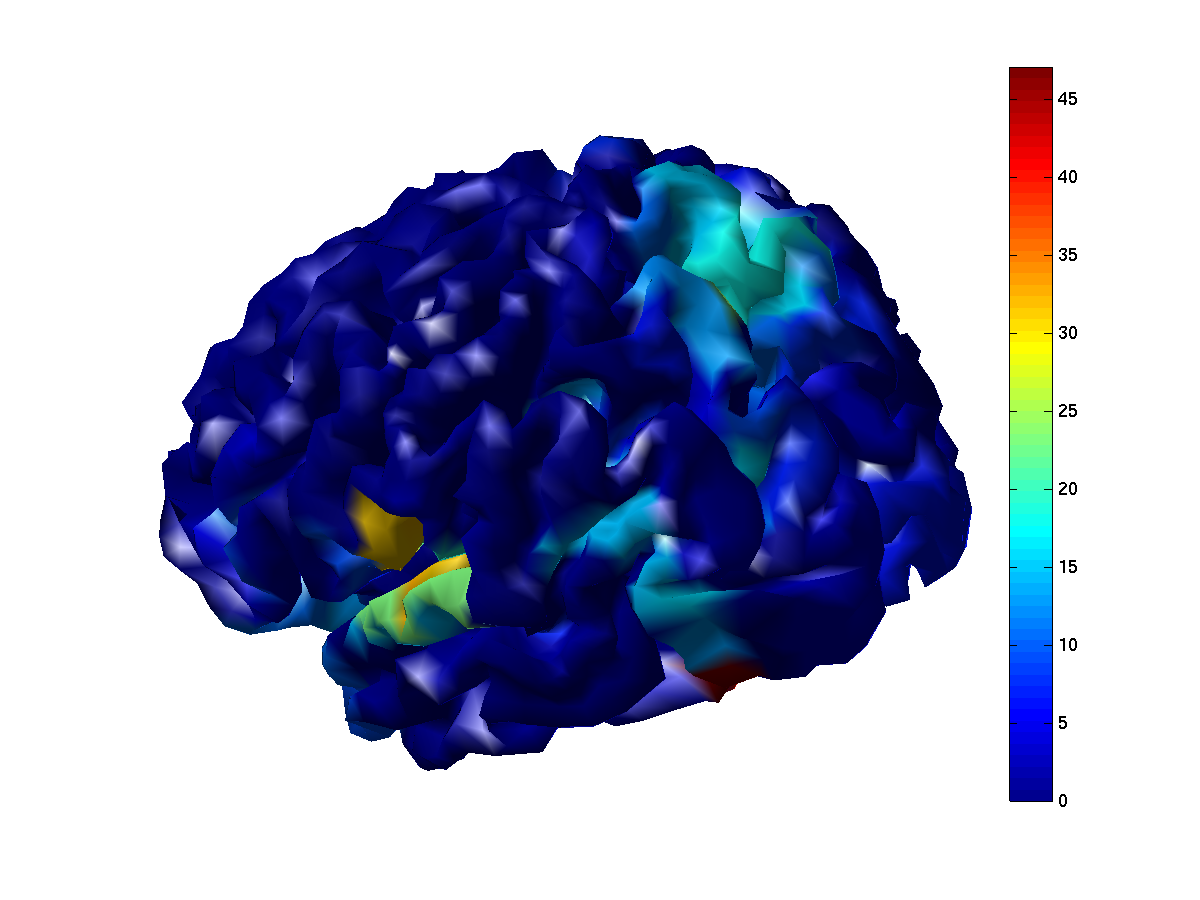Figure 8: Node degree based on imaginary part of coherency, thresholded at a value of 0.1. Cold colors indicated few suptrathreshold connections, warm colors indicate many suprathreshold connections. Left panel: degree based on the thresholded full connectome. Right panel: degree based on the thresholded parcellated connectome.

Compare the degree values for the parcellated and the full connectomes. Why are the values different? What determines the maximum value?

Explore and compare both figures a bit more by 3D rotation. Identify the overlap and discrepancies. What could cause this?

Re-compute the node degree based on some other threshold(s), and inspect the effect of threshold on the result.

Re-compute the parcellated connectome using cfg.method = ‘max’, and inspect the effect of this parameter on the result.

### Exploration of the connectomes in more detail

The graph-based analysis illustrated above allows for only a crude inspection of the connectomes. One detail that is not visualized in this way is the spatial structure of the connections for a given node/parcel. To get a feel how the estimated connectivity patterns change as a function of ‘seed’ location is important. You will notice that the patterns may quite dramatically change, when moving from one seed location to the next. On the other hand, often nearby seed locations will lead to very similar spatial pattern. The directory that contains the data for this tutorial contains a simple function that allows for this exploration. It can be invoked as follow

``````load sourcemodel_4k_inflated;
source_conn.pos = sourcemodel.pos;
tutorial_nwa_connectivityviewer(source_conn, 'cohspctrm', [0 0.1]);
``````

The first input argument is the data structure with the connectivity matrix you want to explore. The second input argument is a string that designates the name of the field to be visualized. The third input argument defines the limits of the color scale. When clicking on the cortical sheet in the figure, you will specify the seed location from which the spatial pattern of connectivity will be displayed.

Invoke the function and explore the data.

### Effect of occipital alpha power on the connectivity results

Compute the connectomes separately on the subsets of trials with low and high occipital alpha power, respectively and inspect the results.

### Using other connectivity metrics

Obviously, one can choose from a large amount of different connectivity measures, each of which has its advantages and disadvantages.

Compute the phase locking value between all pairs of dipoles, as well as a parcellated version. Explore the results, and compare them with the imaginary part of coherency.

Compute the envelope correlations using the ‘powcorr’ method, as well as a parcellated version. Explore the results.